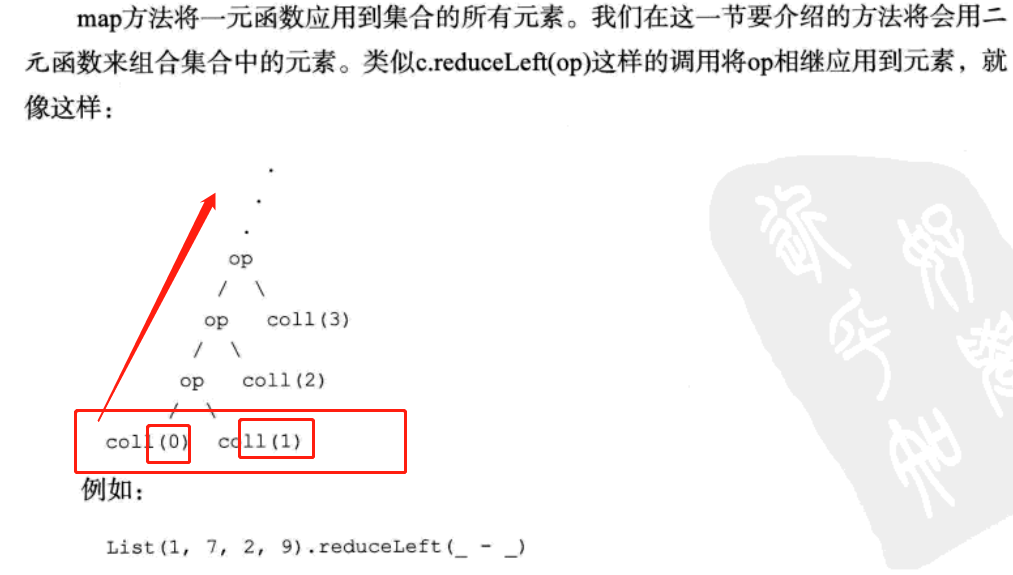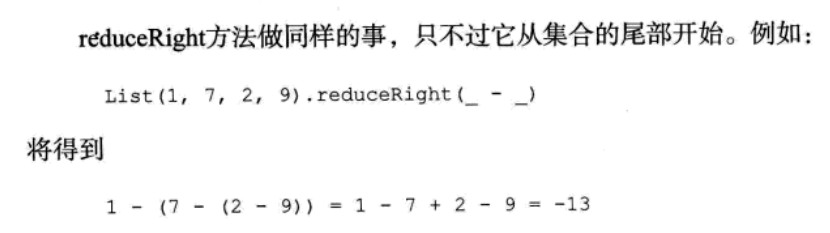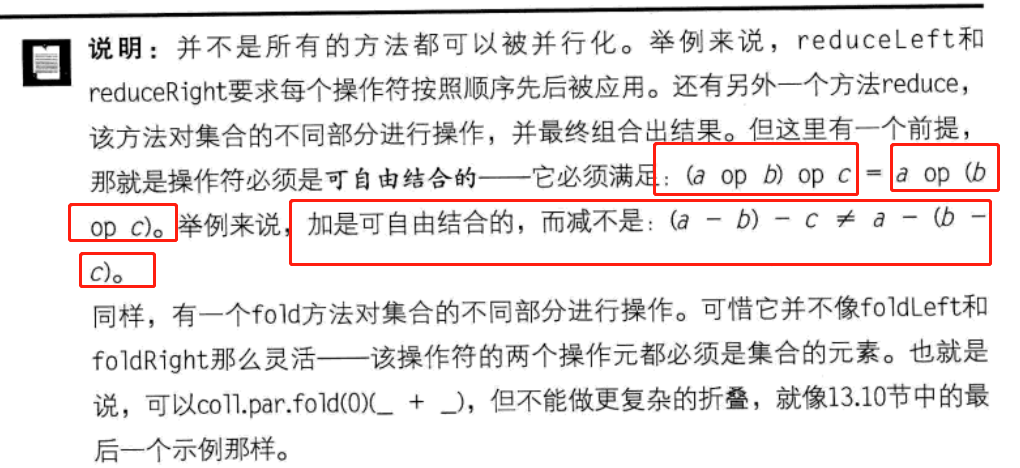# Scala入门reduce操作

categories:资料  author:

reduce包含reduceLeft和reduceRight两种操作，前者从集合的头部开始操作，后者从集合的尾部开始操作。1. scala> val list = List(1,2,3,4,5)
2. list: List[Int] = List(1, 2, 3, 4, 5)
3. scala> list.reduceLeft(_ + _)
4. res21: Int = 15
5. scala> list.reduceRight(_ + _)
6. res22: Int = 15
scala

``````1+2 = 3
3+3 = 6
6+4 = 10
10+5 = 15
``````

reduceRight(_ + _)表示从列表尾部开始，对两两元素进行求和操作，顺序如下：

``````4+5 = 9
3+9 = 12
2+12 = 14
1+14 = 15``````

``````scala> val list = List(1,2,3,4,5)
list: List[Int] = List(1, 2, 3, 4, 5)
scala> list.reduceLeft(_ - _)
res25: Int = -13
scala> list.reduceRight(_ - _)
res26: Int = 3
``````

``````scala> val list = List(1,2,3,4,5)
list: List[Int] = List(1, 2, 3, 4, 5)
scala> list.reduce(_ - _)
res29: Int = -13 //可以看出，得到的结果和reduceLeft的结果是一样的----------
``````

#### Solution

``````scala> val a = Array(12, 6, 15, 2, 20, 9)
a: Array[Int] = Array(12, 6, 15, 2, 20, 9)

scala> a.reduceLeft(_ + _)
res32: Int = 64``````

``````scala> a.reduceLeft(_ * _)
res33: Int = 388800

scala> a.reduceLeft(_ min _)
res34: Int = 2

scala> a.reduceLeft(_ max _)
res35: Int = 20``````
##### Show each step in the process

``````val findMax = (x: Int, y: Int) => {
val winner = x max y
println(s"compared \$x to \$y, \$winner was larger")
winner
}``````
``````scala> a.reduceLeft((x,y) => findMax(x,y))
compared 12 to 6, 12 was larger
compared 12 to 15, 15 was larger
compared 15 to 2, 15 was larger
compared 15 to 20, 20 was larger
compared 20 to 9, 20 was larger
res38: Int = 20``````

• reduceLeft开始调用findMax方来来比较集合的前两个元素，12和6，findMax方法返回12，因为12>6
• reduceLeft接下来使用第一次调用findMax的返回值12和集合的第三个元素15，调用findMax(12, 15)，因为15>12所以findMax返回15
• reduceLeft接下来用每一步执行的返回值和集合的下一个元素传入findMax方法，返回较大的值，直到遍历完集合的最后一个元素，返回最大值20

``````// you provide the sequence 'seq' and the function 'f'
var result = seq(0)
for (i <- 1 until seq.length) {
val next = seq(i)
result = f(result, next)
}``````

##### Working with other sequences and types

``````scala> val peeps = Vector("al", "hannah", "emily", "christina", "aleka")
peeps: scala.collection.immutable.Vector[String] = Vector(al, hannah, emily, christina, aleka)

scala> peeps.reduceLeft((x,y) => if(x.length >= y.length) x else y)
res5: String = christina``````
##### foldLeft, reduceRight, and foldRight

``````scala> val a = Array(1, 2, 3)
a: Array[Int] = Array(1, 2, 3)

scala> a.reduceLeft(_+_)
res6: Int = 6

scala> a.foldLeft(100)(_+_)
res7: Int = 106

scala> a.foldLeft(200)(_+_)
res8: Int = 206``````

##### The difference between reduceLeft and reduceRight

``````val divide = (x: Double, y: Double) => {
val result = x / y
println(s"divided \$x by \$y to yield \$result")
result
}

scala> def divide(x:Double, y:Double):Double = {
|   val result = x / y
|   println(s"divided \$x by \$y to yield \$result")
|   result
| }
divide: (x: Double, y: Double)Double``````

``````scala> val a = Array(1.0, 2.0, 3.0)

scala> a.reduceLeft((x,y) => divide(x,y))
divided 1.0 by 2.0 to yield 0.5
divided 0.5 by 3.0 to yield 0.16666666666666666
res10: Double = 0.16666666666666666

scala> a.reduceRight((x,y) => divide(x,y))
divided 2.0 by 3.0 to yield 0.6666666666666666
divided 1.0 by 0.6666666666666666 to yield 1.5
res11: Double = 1.5``````
##### scanLeft and scanRight

``````scala> def product(x:Int, y:Int):Int = {
|   val result = x * y
|   println(s"multiplied \$x by \$y to yield \$result")
|   result
| }
product: (x: Int, y: Int)Int

scala> val a = Array(1,2,3)
a: Array[Int] = Array(1, 2, 3)

scala> a.scanLeft(10)(product)
multiplied 10 by 1 to yield 10
multiplied 10 by 2 to yield 20
multiplied 20 by 3 to yield 60
res12: Array[Int] = Array(10, 10, 20, 60)``````

``````scala> def findMax(x:Int, y:Int):Int = {
|   val winner = x max y
|   println(s"compared \$x and \$y, the winner is \$winner")
|   winner
| }
findMax: (x: Int, y: Int)Int

scala> val a = Array.range(0,50)
a: Array[Int] = Array(0, 1, 2, 3, 4, 5, 6, 7, 8, 9, 10, 11, 12, 13, 14, 15, 16, 17, 18, 19, 20, 21, 22, 23, 24, 25, 26, 27, 28, 29, 30, 31, 32, 33, 34, 35, 36, 37, 38, 39, 40, 41, 42, 43, 44, 45, 46, 47, 48, 49)

scala> a.reduce(findMax)
compared 0 and 1, the winner is 1
compared 1 and 2, the winner is 2
compared 2 and 3, the winner is 3
compared 3 and 4, the winner is 4
compared 4 and 5, the winner is 5
compared 5 and 6, the winner is 6
compared 6 and 7, the winner is 7
compared 7 and 8, the winner is 8
compared 8 and 9, the winner is 9
compared 9 and 10, the winner is 10
compared 10 and 11, the winner is 11
compared 11 and 12, the winner is 12
compared 12 and 13, the winner is 13
compared 13 and 14, the winner is 14
compared 14 and 15, the winner is 15
compared 15 and 16, the winner is 16
compared 16 and 17, the winner is 17
compared 17 and 18, the winner is 18
compared 18 and 19, the winner is 19
compared 19 and 20, the winner is 20
compared 20 and 21, the winner is 21
compared 21 and 22, the winner is 22
compared 22 and 23, the winner is 23
compared 23 and 24, the winner is 24
compared 24 and 25, the winner is 25
compared 25 and 26, the winner is 26
compared 26 and 27, the winner is 27
compared 27 and 28, the winner is 28
compared 28 and 29, the winner is 29
compared 29 and 30, the winner is 30
compared 30 and 31, the winner is 31
compared 31 and 32, the winner is 32
compared 32 and 33, the winner is 33
compared 33 and 34, the winner is 34
compared 34 and 35, the winner is 35
compared 35 and 36, the winner is 36
compared 36 and 37, the winner is 37
compared 37 and 38, the winner is 38
compared 38 and 39, the winner is 39
compared 39 and 40, the winner is 40
compared 40 and 41, the winner is 41
compared 41 and 42, the winner is 42
compared 42 and 43, the winner is 43
compared 43 and 44, the winner is 44
compared 44 and 45, the winner is 45
compared 45 and 46, the winner is 46
compared 46 and 47, the winner is 47
compared 47 and 48, the winner is 48
compared 48 and 49, the winner is 49
res13: Int = 49``````
`` ``